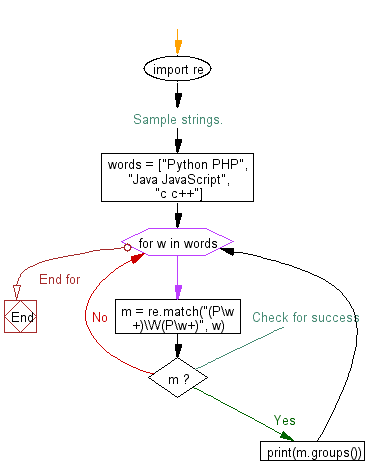﻿ Python Exercises: Match if two words from a list of words starting with letter 'P' - w3resource# Python: Match if two words from a list of words starting with letter 'P'

## Python Regular Expression: Exercise-26 with Solution

Write a Python program to match if two words from a list of words starting with letter 'P'.

Sample Solution:-

Python Code:

``````import re

# Sample strings.
words = ["Python PHP", "Java JavaScript", "c c++"]

for w in words:
m = re.match("(P\w+)\W(P\w+)", w)
# Check for success
if m:
print(m.groups())
```
```

Sample Output:

```('Python', 'PHP')
```

Flowchart:## Visualize Python code execution:

The following tool visualize what the computer is doing step-by-step as it executes the said program:

Python Code Editor:

Have another way to solve this solution? Contribute your code (and comments) through Disqus.

What is the difficulty level of this exercise?

﻿